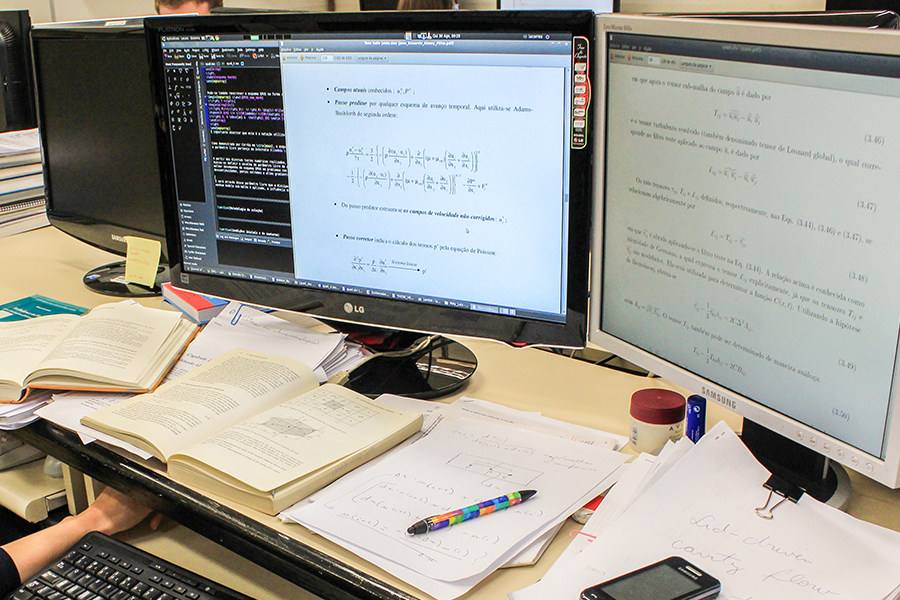NUMBER OF PLACES: 25
LENGTH: 4 years (day classes)The Bachelor´s degree in Applied Mathematics and Scientific Computing is a program in the area of exact sciences focused on the application of mathematical, statistical and computational techniques in solving problems in different areas, such as Engineering, Medicine, Agriculture, Statistics and Finance, among others.

The required course subjects provide a solid background in Mathematics and Computer Programming. The specific courses are designed to train the student in Modeling, Simulations, Statistical Analysis and/or Optimization of Applied Problems. By doing this course, you can choose a balanced education or specialize more by choosing one of these three majors: Optimization; Computational Fluid Mechanics; or Statistics.

WHAT WILL YOU STUDY?

Are you in doubt between Math and Computing? You do not have to choose, you can do both. Simply choose this program option, in which you will have a foundation in Math and Computer Programming, doing courses such as Calculus, Physics, Computer Graphics and Object-oriented Programming. You could also specialize in one of these areas by choosing a number of courses: Statistics; Computational Fluid Mechanics or Optimization.

WHAT CAN YOU DO?

The result of this combination is that you will be able to solve real world real problems by employing or creating mathematical models, using the computer as a working tool. This means you can work anywhere: in industry, software companies, banks and financial institutions, or in public and private research centers. You will be able to transform the problems you face in these places into mathematical models and then solve them.

### CONECT WITH US

© 2023 Instituto de Ciências Matemáticas e de Computação